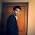# R Function : Gain and Lift Table

This tutorial demonstrates how to calculate gain and lift chart with R. Gain and Lift charts are used to measure the performance of a predictive classification model. They measure how much better results one can expect with the predictive classification model comparing without a model. The lift metrics is a very popular metrics to evaluate the customer and marketing analytics model. The 'lift' term comes from marketing analytics domain.

R Function : Gain and Lift Chart
lift <- function(depvar, predcol, groups=10) {
if(!require(dplyr)){
install.packages("dplyr")
library(dplyr)}
if(is.factor(depvar)) depvar <- as.integer(as.character(depvar))
if(is.factor(predcol)) predcol <- as.integer(as.character(predcol))
helper = data.frame(cbind(depvar, predcol))
helper[,"bucket"] = ntile(-helper[,"predcol"], groups)
gaintable = helper %>% group_by(bucket)  %>%
summarise_at(vars(depvar), funs(total = n(),
totalresp=sum(., na.rm = TRUE))) %>%
mutate(Cumresp = cumsum(totalresp),
Gain=Cumresp/sum(totalresp)*100,
Cumlift=Gain/(bucket*(100/groups)))
return(gaintable)
}

Run Function
dt = lift(churn\$target , churn\$prediction, groups = 10)
In the first parameter of the lift function, you need to define a column wherein binary target variable (dependent variable) is stored. In this case, churn\$target : churn is the data frame and target is the dependent variable column.

In the second parameter, you need to specify the predicted probability variable.

How to plot Cumulative Lift

Run the following code to create lift chart.
graphics::plot(dt\$bucket, dt\$Cumlift, type="l", ylab="Cumulative lift", xlab="Bucket")Lift Chart
Interpretation of Cumulative Lift
The Cumulative Lift of 3.4 for top two deciles, means that when selecting 20% of the records based on the model, one can expect 3.4 times the total number of targets (events) found by randomly selecting 20%-of-records without a model. In terms of customer attrition (churn) model, we can say we can cover 3.4 times the number of attritors by selecting only 20% of the customers based on the model as compared to 20% customer selection randomly.

Output

The output of gain and lift table is shown in the image below -Gain and Lift Table
Interpretation of Gain
% of targets (events) covered at a given decile level. For example,  67.9% of events covered in top 20% of data based on model.

Export gain and lift table

You can export gain table in CSV format by submitting the following code -
# Export gain table
write.csv(dt, "D:\\lift.csv", row.names = FALSE)
Related Tutorial - Understand Gain and Lift Chart#### R Tutorials : 75 Free R Tutorials

About Author:Deepanshu founded ListenData with a simple objective - Make analytics easy to understand and follow. He has over 7 years of experience in data science and predictive modeling. During his tenure, he has worked with global clients in various domains like banking, Telecom, HR and Health Insurance.

While I love having friends who agree, I only learn from those who don't.

Let's Get Connected: Email | LinkedIn

Get Free Email Updates :
Related Posts:
8 Responses to "R Function : Gain and Lift Table"
1.2.Thanks for the code. However, after running it, the gain table that I am getting, its not dividing the data into 10 equal groups, each group is having different observation number, ideally which should not be the case. Need help on this.

1.It is because some of predicted probability scores are equal so one or two groups are not of same size. I am assuming the difference of number of observations in each group is very minimal. For example, c(0.1,0.2,0.3,0.3,0.4,0.5) - you cannot assign different rank to 0.3 in this vector.

To have same observations in each group, you can add ties.method = "first" after groups = 10 in the code. Revised code would be -
gaint = gains(actual= valgain[,1], predicted=pred ,groups=10, ties.method = "first")

Hope it helps!

3.I keep getting this error:
Error in `[.data.frame`(helper, , "predcol") : undefined columns selected
Calls: lift -> ntile -> [ -> [.data.frame

I'm not able to understand why.

PS I love this post!

4.Wt is depvar and predcol pls explain

1.depvar : Dependent Variable
predcol : Predicted Probability column

5.Hi, I am keep getting this error when trying to create a table with lift function: "error in lift.default: 'x' should be a formula". Would you be able to help me with it?

6.How to get which customers are in top 3 deciles from gains plot? I mean how to retrieve those observations from top deciles?

Next → ← Prev